# Test: Fault Analysis of Power Systems

## 10 Questions MCQ Test Topicwise Question Bank for Electrical Engineering | Test: Fault Analysis of Power Systems

Description
Attempt Test: Fault Analysis of Power Systems | 10 questions in 30 minutes | Mock test for Electrical Engineering (EE) preparation | Free important questions MCQ to study Topicwise Question Bank for Electrical Engineering for Electrical Engineering (EE) Exam | Download free PDF with solutions
QUESTION: 1

### When all the three phases are short circuited it gives rise to

Solution:

A three-phase fault is a symmetrical fault.

QUESTION: 2

### The zero sequence reactance will be more than the positive sequence reactance, if the equipment is:

Solution:

For transmission line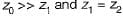QUESTION: 3

### The sequence networks for a three-phase faults are connected in

Solution:

A three-phase fault is a symmetrical fault where only positive sequence network (current exists).

QUESTION: 4

Match List-l (Symbol for 3-phase transformer connections) with List-ll (Zero-sequence equivalent circuits) and select the correct answer using the codes given below the lists:
List-I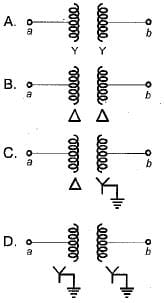List-ll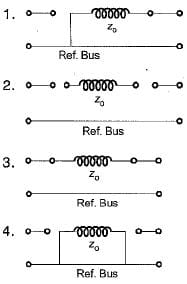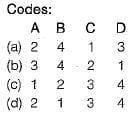Solution:

Using switch diagram, we find the zero sequence network for a transformer.

QUESTION: 5

The base impedance and base voltage for a given power system are 10Ω and 400 V respectively. The base kVA and the base current will be respectively

Solution: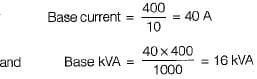QUESTION: 6

The per-unit admittance YPu of a power system in terms of the base voltage Vbase and the base voltamperes (VA)base is given by

Solution: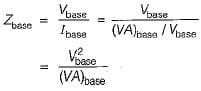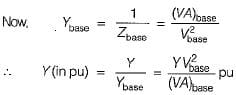QUESTION: 7

In a line to ground fault on a system, the fault current is 1500 A. The zero sequence current is

Solution: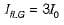or,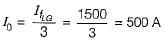QUESTION: 8

The fault current, in a double line to ground fault on a system depends on

Solution: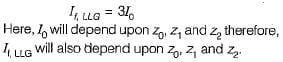QUESTION: 9

There may be a zero sequence components in
1. line voltage of star circuit
2. phase voltage of star circuit
3. line current of delta circuit
4. phase current of delta circuit
Select the correct answer from the given options:

Solution:
QUESTION: 10

A 10 kVA, 400 V/200V single phase transformer with 10% impedance draws a steady short circuit line current of

Solution: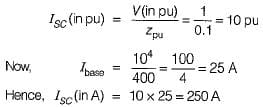Use Code STAYHOME200 and get INR 200 additional OFF Use Coupon Code